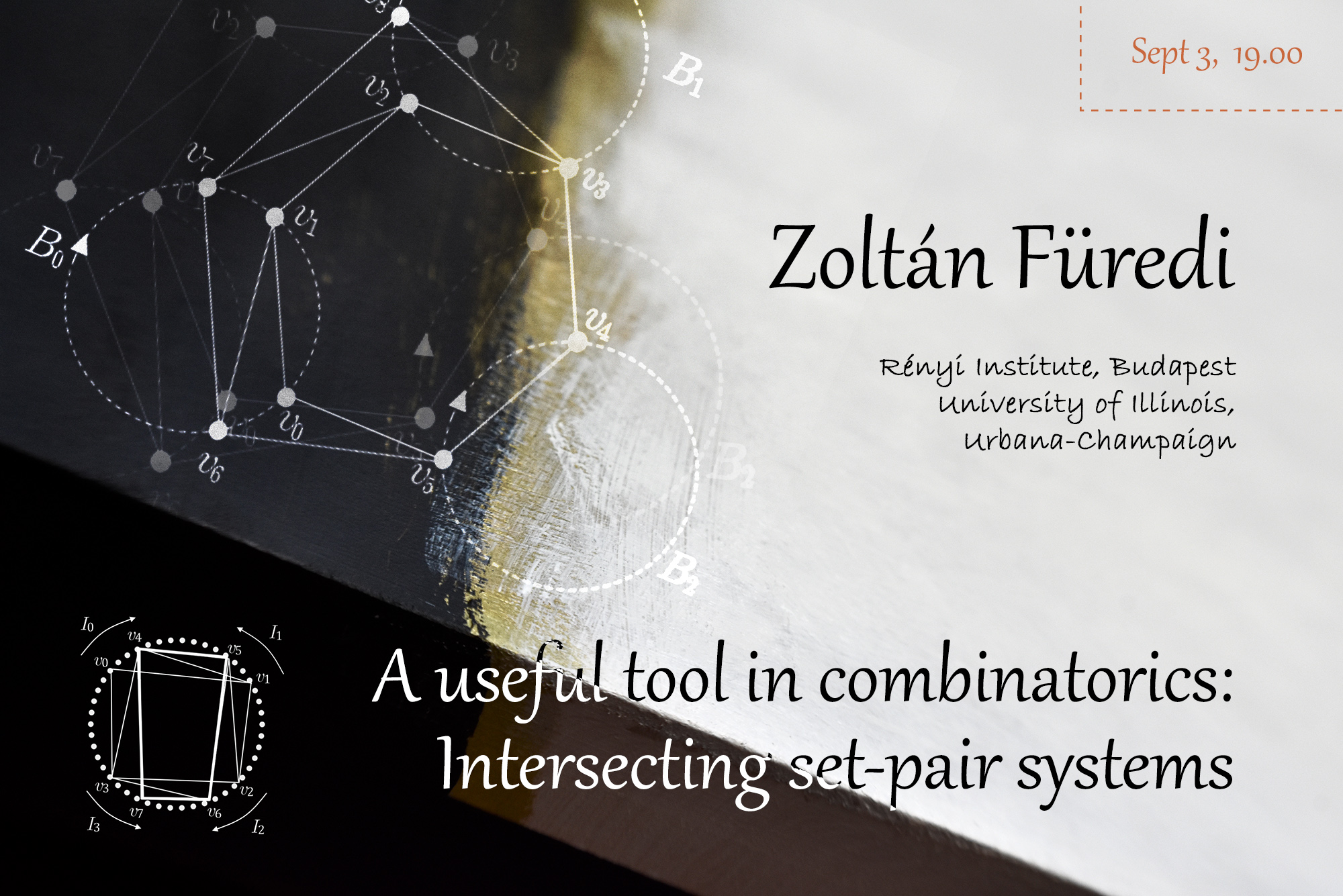# Zoltán Füredi “A useful tool in combinatorics: Intersecting set-pair systems”September 3, 2020
19.00 MSK (UTC +3)

# Zoltán Füredi "A useful tool in combinatorics: Intersecting set-pair systems"

Zoltán Füredi from Rényi Institute and University of Illinois will give the talk "A useful tool in combinatorics: Intersecting set-pair systems" on the labs' Big Seminar.

The talk will be held in zoom
Meeting ID: 279-059-822
Password: first 6 decimal places of $\pi$ after the decimal point

You can also write to Alexander Polyanskii (alexander.polyanskii@yandex.ru) or to Maksim Zhukovskii (zhukmax@gmail.com) if you want to be added to mailing list.

Abstract:

The notion of cross intersecting set pair system (SPS) of size $m$, $\Big(\{A_i\}_{i=1}^m, \{B_i\}_{i=1}^m\Big)$ with $A_i\cap B_i=\emptyset$ and $A_i\cap B_j\ne\emptyset$, was introduced by Bollobás and it became an important tool of extremal combinatorics. His classical result states that $m\le {a+b\choose a}$ if $|A_i|\le a$ and $|B_i|\le b$ for each $i$.

After reviewing classical proofs, applications and generalizations, our central problem is to see how this bound changes with additional conditions.

In particular we consider $1$-cross intersecting set pair systems, where $|A_i\cap B_j|=1$ for all $i\ne j$. We show connections to perfect graphs, clique partitions of graphs, and finite geometries. Many problems are proposed. Most new results is a joint work with A. Gyárfás and Z. Király.

## Watch the video:

Everyone is invited to attend. The language of the lecture is English. The event is aimed at master and graduate students, as well as researchers in the field of combinatorics.